The REG Procedure
 Testing for Lack of Fit

The test for lack of fit compares the variation around the model with "pure" variation within replicated observations. This measures the adequacy of the specified model. In particular, if there are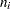replicated observations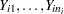of the response all at the same values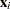of the regressors, then you can predict the true response ateither by using the predicted value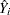based on the model or by using the mean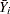of the replicated values. The test for lack of fit decomposes the residual error into a component due to the variation of the replications around their mean value (the "pure" error) and a component due to the variation of the mean values around the model prediction (the "bias" error):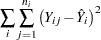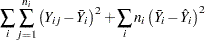If the model is adequate, then both components estimate the nominal level of error; however, if the bias component of error is much larger than the pure error, then this constitutes evidence that there is significant lack of fit.

If some observations in your design are replicated, you can test for lack of fit by specifying the LACKFIT option in the MODEL statement (see Example 74.6). Note that, since all other tests use total error rather than pure error, you might want to hand-calculate the tests with respect to pure error if the lack of fit is significant. On the other hand, significant lack of fit indicates that the specified model is inadequate, so if this is a problem you can also try to refine the model.Previous Page | Next Page | Top of Page